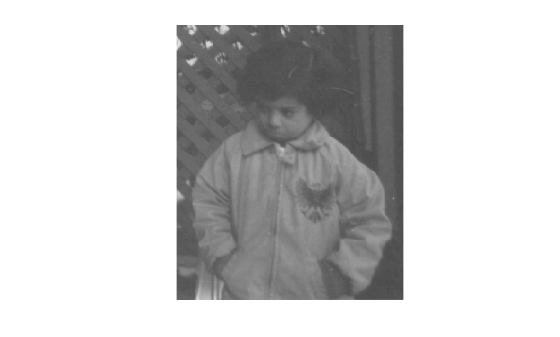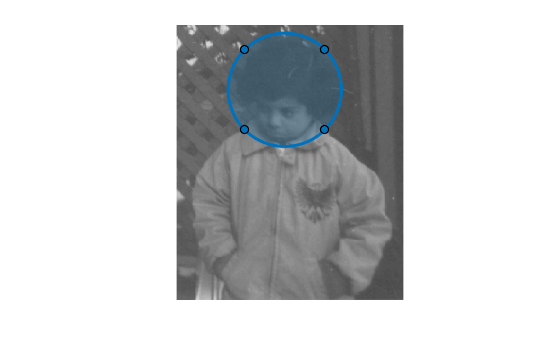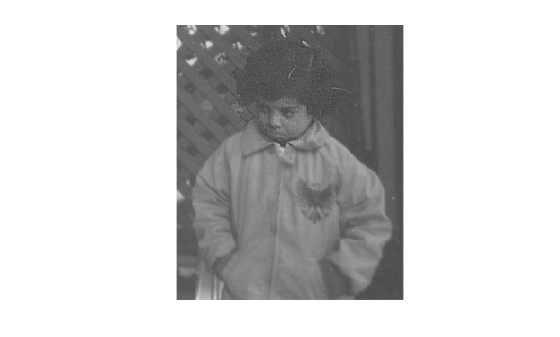# Sharpen Region of Interest in an Image

Read a grayscale image into the workspace.

```I = imread('pout.tif'); imshow(I)```Draw a region of interest over the image to specify the area you want to filter. Use the `drawcircle` function to create the region of interest, specifying the center of the circle and the radius of the circle. Alternatively, if you want to draw the circle interactively, then do not specify the center or radius of the circle.

`hax = drawcircle(gca,'Center',[115 69],'Radius', 60);`Create the mask using the `createMask` function and specifying the ROI.

`mask = createMask(hax);`

Define the function you want to use as a filter. This function, named `f`, passes the input image `x` to the `imsharpen` function and specifies the strength of the sharpening effect by using the `'Amount'` name-value pair argument.

`f = @(x)imsharpen(x,'Amount',3)`
```f = function_handle with value: @(x)imsharpen(x,'Amount',3) ```

Filter the ROI using the `roifilt2` function and specifying the image, mask, and filtering function.

`J = roifilt2(I,mask,f);`

Display the result.

`imshow(J)`